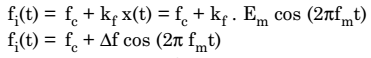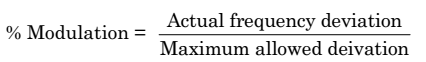# Define Modulation Index,Frequency Deviation and Percent Modulation For FM Wave

## Modulation Index of FM Wave

The modulation index of an FM wave is defined as under:The modulation index (mf) is very important in FM as it decides the bandwidth of the FM wave . The modulation index also decides the number of side bands having significant amplitudes. In AM, the maximum value of the modulation index m is 1. But, for FM, the modulation index can be greater than 1.

## Frequency Deviation (Δf) of FM Wave

For the single tone FM i.e., the modulating signal x(t) be a sinusoidal signal of amplitude Em and frequency fm.

Therefore,The unmodulated carrier is represented by the expression:In FM, the frequency f of the FM wave varies in accordance with the modulating voltage. Thus,Where, Δf = kf Eand it is called as frequency deviation.

Frequency deviation (Δf) represents the maximum departure of the instantaneous frequency fi(t) of the FM wave from the carrier frequency fc.

Since Δf = kf Em , the frequency deviation is proportional to the amplitude of modulating voltage (Em) and it is independent of the modulating frequency fm.

## Percent Modulation of FM Wave

The percent modulation is defined as the ratio of the actual frequency deviation produced by the modulating signal to the maximum allowable frequency deviation.

Thus,